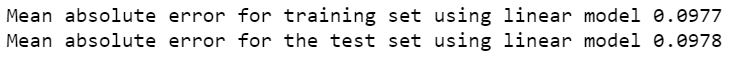# Kaggle PUBG Competition Building a Model

Having completed our analysis for the Player Unknown Battlegrounds dataset from Kaggle we can now build a model. We can start by building a very simple linear regression model as a baseline. We have already established that at least some of the parameters have nonlinear relations. However a linear model gives us an idea of the minimum level of accuracy we should accept.

Lets build our models with the Scikit Learn library since it has a wide range of algorithms to choose from. First, lets try a basic linear model with no engineered features. Lets use only those variable which seemed to have high correlation with our target variable. So that we can iterate fast, lets split out a validation set from the training data. This will also allow us to examine errors as we have access to the target value for the training set. Here is a basic linear model

```
# machine learning imports
import sklearn as skl
import pandas as pd
from sklearn.linear_model import LinearRegression # does not auto import
from sklearn.metrics import mean_absolute_error # does not auto import
import numpy as np

# import our data
# there is a NaN value we need to drop
pubg_data = pubg_data.dropna()

# select our features
labels = ['boosts', 'damageDealt', 'heals', 'killPlace', 'kills',
'killStreaks', 'longestKill', 'revives', 'rideDistance', 'walkDistance',
'weaponsAcquired']

# create input data
pubg_x = pubg_data[labels]

# set up our target data
pubg_y = pubg_data['winPlacePerc']

# now lets scale data
scaler = skl.preprocessing.StandardScaler().fit(pubg_x)

# lets look at the head again
# we need to convert back to dataframe from numpy array though
pubg_x = pd.DataFrame(scaler.transform(pubg_x), columns= labels)

# partition into train and test
pubg_x_train, pubg_x_test, pubg_y_train, pubg_y_test = (
skl.model_selection.train_test_split(pubg_x, pubg_y, random_state = 9))

# now lets create the model
model = LinearRegression()

# and fit it...
model.fit(pubg_x_train, pubg_y_train);

# now lets test how well it fits training data and unseen data
predict_train = model.predict(pubg_x_train)
print('Mean absolute error for training set using linear model %.4f'
% mean_absolute_error(pubg_y_train, predict_train))
predict_test = model.predict(pubg_x_test)
print('Mean absolute error for the test set using linear model %.4f'
% mean_absolute_error(pubg_y_test, predict_test))
```

This model actually performs surprisingly well. Its mean absolute error (the metric being used in the competition) is only 10%.This is especially surprising considering:

• the lack of feature engineering
• the extreme value clipping
• the fact that Scikit Learn’s Linear Regression works on mean squared error.

We can now iterate on this model to explore whether clipping extreme input and output variables will be beneficial. Lets clip the inputs first

```
# clip outliers on a per column basis
pubg_x = pubg_x.clip(lower=None, upper= pubg_x.quantile(0.999), axis = 1)

```

And also we can clip the outputs, currently we shall do this just at the evaluation stage. Later we can build it into our postprocessing of the model if it seems effective. We can clip the inputs with Panda’s clip function. However the outputs from SciKit Learn are Numpy arrays and need to be clipped with different syntax

```
# updated accuracy estimators
print('Mean absolute error for training set using linear model %.4f'
% mean_absolute_error(pubg_y_train, np.clip(predict_train,0,1)))
predict_test = model.predict(pubg_x_test)
print('Mean absolute error for the test set using linear model %.4f'
% mean_absolute_error(pubg_y_test, np.clip(predict_test,0,1)))

```

This reduces our error only slightly, but suggests that clipping is worthwhile.After this we can introduce our feature engineering algorithm and select from our engineered features looking for a good combination. Once we have a good set of features, it is time to move on to a more sophisticated model. We need to pick a model which can make use of the non-linear relationships we found in our data. For this example I am choosing to use a Random Forest Regressor.

The feature engineering code was covered in the last post. The broad sweep of setting up and running a SciKit Learn model is shown by the simple example above. Therefore I shall not insert the code in this post.  You can obtain a copy of the finished model here. Instead I shall discuss optimisation strategy.

Initially the classifier was run against the same validation set as the linear model with a small number of trees. This allowed for rapid iteration to test different combinations of input features. During this process I made use of OOB error estimates and also of the feature importances for the model which can be obtained via:

```
RandomForestRegressor.feature_importances_

```

This allowed me to check which features were proving most useful in training the tree. This turned out to be the features previously identified, normalised with respect to the averages in the relevant match. It was also the same variables but normalised as group averages. Features comprising head shot to kill ratios and kill streaks to kill ratios also proved useful, likely because these metrics help estimate player skill. Finally I used some information about game type from just a few one shot encoded game type indicator variables. This likely helps because different game modes require slightly different balances of skills and reflected in different weightings for the features.

Once all this was done and I had a model I was happy with, I increased the number of trees and trained the model on the entire training set, then used it to fit the test set. Final result: a mean absolute error below 5%. Not bad for a comparatively simple model.As usual Jupyter notebooks can be found on my GitHub account.

This site uses Akismet to reduce spam. Learn how your comment data is processed.